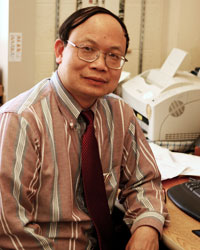## Keynote SpeakerProf. Sun-Yuan Kung

Princeton University, USA

Title: Supervised and Unsupervised Deep Learning: Theory and Applications

Abstract: Two most successful neural networks for machine learning are MLPs and CNNs. What setting CNNs apart from MLPs is that it makes a good use of the kernel diversity. Namely, the elementary multiplication module in MLPs are being upgraded to the more powerful kernel, i.e. convolution, building blocks for CNNs. Indeed, kernel diversity has brought about enormous successes in many real-world applications. As such, it is widely regarded as the hallmark of deep learning. In this talk, we shall explore the fundamental theory concerning kernel diversity, which will in turn further advance our neural architectural design strategies.

Theoretically, we first investigate both supervised and unsupervised deep learning paradigms:

• Supervised Deep Learning: The classic LSE problem has to do with optimally projecting the input space onto output space, i.e. $$y(t) ≅ Wx(t)$$. The optimization process involves minimization of the ensemble LSE error: find $$W$$ for $$Min \sum_t||y(t)–Wx(t)||^2$$. Its Deep LSE variant involves minimization of ensemble DLSE error, i.e. $$Min\sum_t||y(t)–W(t) ∗ x(t)||^2$$. For speeches, $$W(t)$$ is simply a polynomial matrix of a time index $$t$$. For image feature maps, on the other hand, it represents a two-spatial-variable polynomial matrix: $$W(t) = W(t_1, t_2)$$.
• Unsupervised Deep Learning: Two such learning paradigms will be presented: Deep-PCA and Deep K-means. Deep-PCA is basically a low-rank variant of DLSE, with the polynomial matrix $$W(t)$$ being confined to a small rank. As to Deep K-means, we note that LSE itself may serve well as a distance metric between the two (normalized) feature maps $$x(t)$$ and $$y(t)$$. Thanks to the diversity gain, it is mathematically assured that DLSE ≤ LSE. As a result, one might resort to the kernel modulated metric, $$d(x(t),y(t)) = < x(t),y(t) >_{W(t)}$$, to better calibrate the similarity between two feature maps. Such a metric ultimately leads to deep clustering methods, exemplified by Deep K-means and Deep Spectral clustering.

The proposed learning paradigms may prove handy for Neural Architecture Search (NAS) which stands for a design software system for automatically learning the optimal parameters and optimal structures of deep learning networks. We shall highlight two such design examples:

• Deep-PCA and its variants may prove handy to both Regressive NAS (RNAS) and Progressive NAS (PNAS) design strategies. Some illuminating applications, including neural networks with improved classification and/or enhanced super-resolution images, will be showcased.
• As another example, our simulation study shows that a proper combination of DCA-based knowledge distillation and hierarchical clustering can yield the best RNAS design.

Bio: S.Y. Kung, Life Fellow of IEEE, is a Professor at Department of Electrical Engineering in Princeton University. His research areas include machine learning, data mining, systematic design of (deep-learning) neural networks, statistical estimation, VLSI array processors, signal and multimedia information processing, and most recently compressive privacy. He was a founding member of several Technical Committees (TC) of the IEEE Signal Processing Society. He was elected to Fellow in 1988 and served as a Member of the Board of Governors of the IEEE Signal Processing Society (1989-1991). He was a recipient of IEEE Signal Processing Society's Technical Achievement Award for the contributions on "parallel processing and neural network algorithms for signal processing" (1992); a Distinguished Lecturer of IEEE Signal Processing Society (1994); a recipient of IEEE Signal Processing Society's Best Paper Award for his publication on principal component neural networks (1996); and a recipient of the IEEE Third Millennium Medal (2000). Since 1990, he has been the Editor-In-Chief of the Journal of VLSI Signal Processing Systems. He served as the first Associate Editor in VLSI Area (1984) and the first Associate Editor in Neural Network (1991) for the IEEE Transactions on Signal Processing. He has authored and co-authored more than 500 technical publications and numerous textbooks including "VLSI Array Processors", Prentice-Hall (1988); "Digital Neural Networks", Prentice-Hall (1993) ; "Principal Component Neural Networks", John-Wiley (1996); "Biometric Authentication: A Machine Learning Approach", Prentice-Hall (2004); and "Kernel Methods and Machine Learning”, Cambridge University Press (2014).